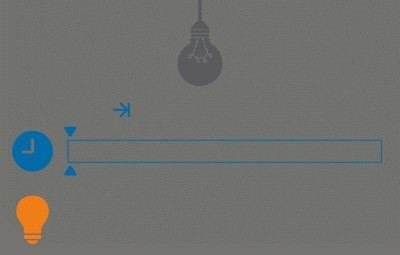Lightbulb Start Watching
Simple
English RU

This is the second mission in the lightbulb series. I will try to make each following task slightly more complex.

You have already learned how to count the amount of time a light bulb has been on, or how long a room has been lit. Now let's add one more parameter - the counting start time.

This means that the light continues to turn on and off as before. But now, as a result of the function, I want not only to know how long there was light in the room, but how long the room was lit, starting from a certain moment.

One more argument is added – start_watching , and if it’s not passed, we count as in the previous version of the program for the entire period.Input: Two arguments and only the first one is required. The first one is a list of datetime objects and the second one is a datetime object.

Output: A number of seconds as an integer.

Example:

```sum_light([
datetime(2015, 1, 12, 10, 0, 0),
datetime(2015, 1, 12, 10, 0, 10),
],
datetime(2015, 1, 12, 10, 0, 5)) == 5

sum_light([
datetime(2015, 1, 12, 10, 0, 0),
datetime(2015, 1, 12, 10, 0, 10),
], datetime(2015, 1, 12, 10, 0, 0)) == 10

sum_light([
datetime(2015, 1, 12, 10, 0, 0),
datetime(2015, 1, 12, 10, 10, 10),
datetime(2015, 1, 12, 11, 0, 0),
datetime(2015, 1, 12, 11, 10, 10),
], datetime(2015, 1, 12, 11, 0, 0)) == 610
```

Precondition:

• The array of pressing the button is always sorted in ascending order
• The array of pressing the button has no repeated elements
• The amount of elements is always even (the light will eventually be off)
• The minimum possible date is 1970-01-01
• The maximum possible date is 9999-12-31